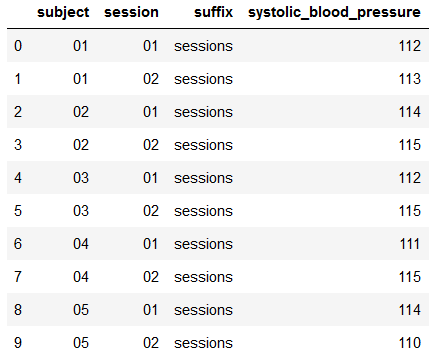# Pybids - layout get_collections does not work for sessions

Hello,

I was experimenting with the methods of the BIDSLayout class using the test examples given in the tutorial and the bids-examples repository.

I initialized my layout:
`layout2 = BIDSLayout(join(get_test_data_path(), 'synthetic'), validate=False, derivatives=True)`

When I try to run:
`layout2.get_collections(level='subject', merge=True).to_df()`, it collects all the tsv files under the subject directory for all the subjects and stores them in a dataframe.However when I try to run:
`layout2.get_collections(level='session', merge=True).to_df()`
it gives me a `KeyError: "['extension'] not found in axis"`.

I tried to dig into the source code and found that it adds an `extension` column to the table.
`E:\Anaconda\lib\site-packages\bids\variables\io.py in _load_tsv_variables(layout, suffix, dataset, columns, prepend_type, scope, **selectors) 432 dupes = list(set(ent_cols.columns) & set(_data.columns)) --> 433 to_drop = ['extension'] + dupes 434 ent_cols.drop(columns=to_drop, inplace=True) 435 436 _data = pd.concat([_data, ent_cols], axis=1, sort=True)`

The session folders also have their own `*scans.tsv` files.

Since this was from the bids-examples dataset, I figured out it might be easier for you guys to test this out as well. Any solutions or suggestions?